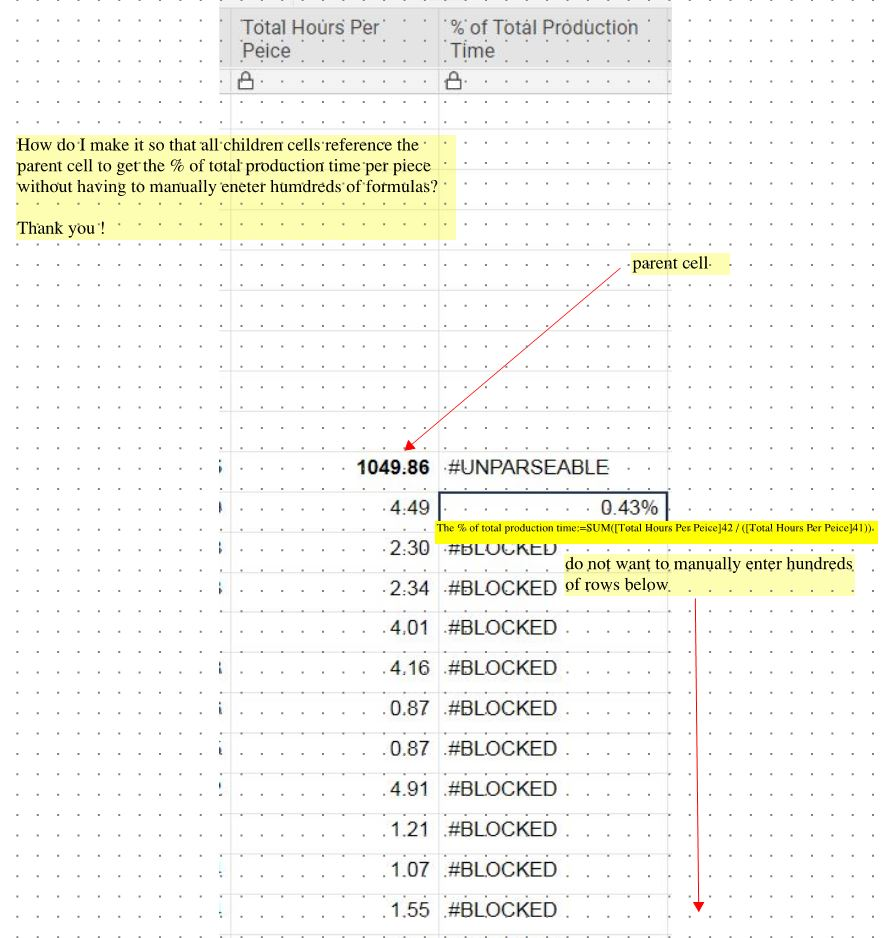# Formula TO NOT have to manully enter formula for every row

Good morning,

Looking for some help on this issue.

We have a sheet with hundreds of rows that tally up to a total (1049.86 hours per below JPEG)

We need to calculate the % each piece is from the total . These are the children rows.

The first formula I got to work by using =SUM([Total Hours Per Peice]42 / ([Total Hours Per Peice]41))

How do I get all the children rows to reference the parent row without having to manually change the formula in every row?

Thank youTags:

• 2 ways.

=[total hours per peice]42 / [total hours per peice]\$41

=[total hours per peice]42/ parent([total hours per peice]42)

• thank you

does the \$ sign mean that it will always reference that cell?

• Sorry , one more question, how do I get it to work so that all the children rows calculate if a new row is created?

thanks

• it means it will always reference that row. if you want to always reference that specific cell you need 2 dollar signs

row and column

\$[Primary Column]\$1

row

[Primary Column]\$1

Column

\$[Primary Column]1

Nothing

[Primary Column]1

• Depends. How are you adding the new rows?

• when we have an add to the job (more scope) we manually enter a new  at the bottom of the sheet, but we are going to also add the ability to do it by a form as well

thanks

• There is only one parent child reference or are you planning on adding to multiple?

• there is only one parent child reference

thanks

• Keep in mind that using a form to add new rows will not pull down formulas if there is any hierarchy in the row above. In other words... If the last row is a child row, the next row entered by a form will not automatically be a child row and will not automatically pull the formula.

• I would get rid of the parent child reference and just use column references instead. It is a lot more straightforward. If you are worried about collapsing your sheet you can build some shared filters to get a similar functionality. a column reference is made by simply deleting the row reference off a range

Ex

[Column Name]:[Column Name]

## Help Article Resources

Want to practice working with formulas directly in Smartsheet?

Check out the Formula Handbook template!